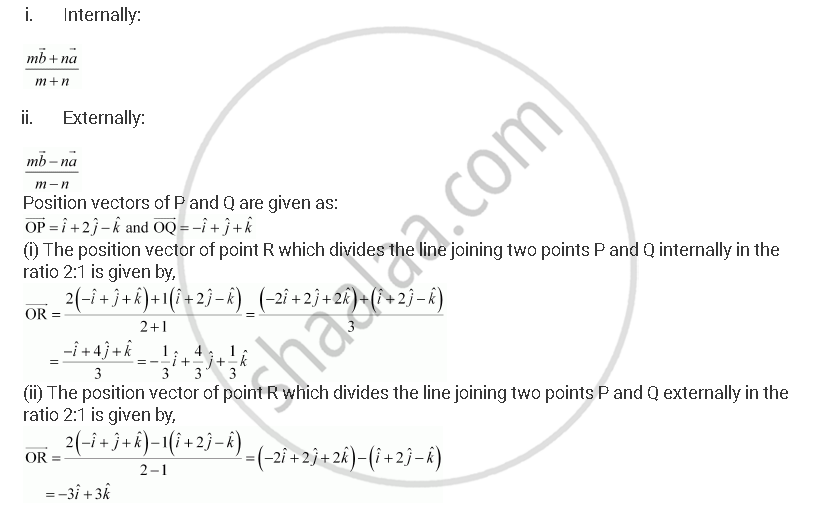Share

# Find the Position Vector of a Point R Which Divides the Line Joining Two Points P and Q Whose Position Vectors Are Hati + 2hatj - Hatk and -hati + Hatj + Hatk Respectively, in the Ration 2:1 - CBSE (Science) Class 12 - Mathematics

ConceptBasic Concepts of Vector Algebra

#### Question

Find the position vector of a point R which divides the line joining two points P and Q whose position vectors are  hati + 2hatj - hatk and -hati + hatj + hatk  respectively, in the ration 2:1

(i) internally

(ii) externally

#### Solution

The position vector of point R dividing the line segment joining two points P and Q in the ratio m: is given by:Is there an error in this question or solution?

#### Video TutorialsVIEW ALL 

Solution Find the Position Vector of a Point R Which Divides the Line Joining Two Points P and Q Whose Position Vectors Are Hati + 2hatj - Hatk and -hati + Hatj + Hatk Respectively, in the Ration 2:1 Concept: Basic Concepts of Vector Algebra.
S